# EXACT Function

Tests if two strings are exactly equal and if so, returns TRUE

## What is the EXACT Function?

The EXACT Function is categorized under Excel TEXT functions. The function helps to test if two or more than two supplied text strings or values are exactly equal and, if so, returns TRUE. Otherwise, it returns FALSE. The EXACT function is case-sensitive.

In financial analysis, we often need to compare two values when analyzing data. The EXACT function helps us to do the comparison. Also, it can be useful when we share our worksheet with others and want them to input data in a specific format.

### Formula

=EXACT(text1, text2, …)

Arguments:

1. Text1 (required argument) – This is the first string which we wish to join. It can be two text strings, numbers, or logical value.
2. Text2 (required argument) – This is the second text string.

The EXACT function returns TRUE if the two strings are the same. The EXACT function returns FALSE if the two strings are different.

### How to use the EXACT Function in Excel?

To understand the uses of this function, let’s consider a few examples:

#### Example 1

Suppose we wish to compare following data:

ZoneZone
NorthNORTH
SouthSouth
Zonezone

The formula used and the result we get is as follows: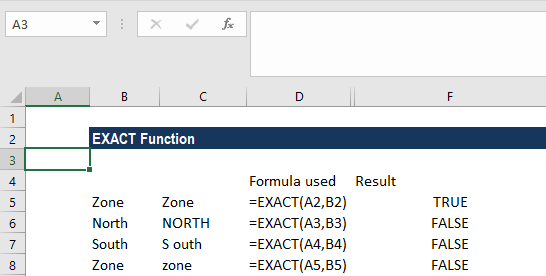Let’s analyze the data:

1. In the first example, the result is true, as the strings match.
2. As the text strings in the next row are different, one is in upper case and the second is in lower case, we get the result as false. As the function is case sensitive, we get the result as FALSE.
3. Similarly, we get false for the next two examples, as they don’t match.

#### Example 2

Suppose we wish that users of our worksheet enter data in PROPER case. Only then we can use data validation with a custom formula based on the EXACT and other functions.

Suppose the data given is as follows: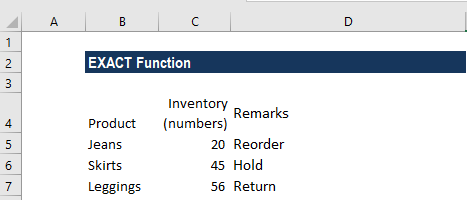We wish any user of this worksheet to input remarks in proper case only. We can then click on data validation under Data tab and select Data Validation. Change the validation criteria to custom and input the formula =AND(EXACT(C5,PROPER(C5)),ISTEXT(C5)).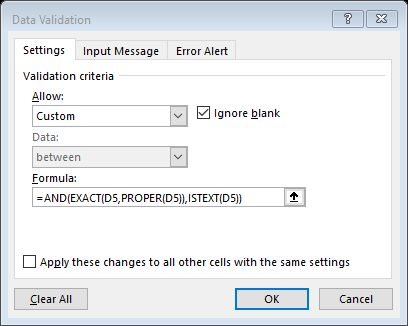Now if any user tries to put any value that is not in the proper case, they will get the following error: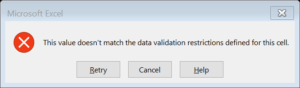How the formula worked:

The Data validation rules we set are triggered when a user adds or changes a cell value. The PROPER function changes text values to proper case, and the EXACT function performs a case-sensitive comparison. The AND function takes multiple arguments (logical conditions) and returns TRUE only when all arguments return TRUE.

So, the first logical condition would compare the value input by the user to a proper case version of the same value EXACT(C3,PROPER(C3) and the second logical condition tests that input to C5 is actually text ISTEXT(C3).

Now if both of the above conditions are true, then the AND function returns TRUE, and the input passes validation. If either condition is FALSE, AND returns FALSE and input fails the data validation.

#### Example 3

The EXACT function is quite useful when we wish to deal with case-sensitive data.

Using the different product details and their revenue for the year: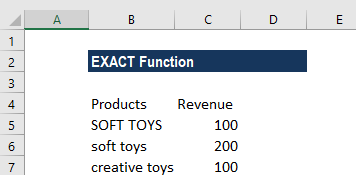As shown above, there are two products, soft toys in lowercase and SOFT TOYS in uppercase. Excel functions such as VLOOKUP and INDEX/MATCH are not case-sensitive. As we are looking out for numeric values, SUMPRODUCT + EXACT is an exciting and flexible way to do a case-sensitive lookup.

The formula to be used is =SUMPRODUCT(–(EXACT(E3,B3:B8)),C3:C8).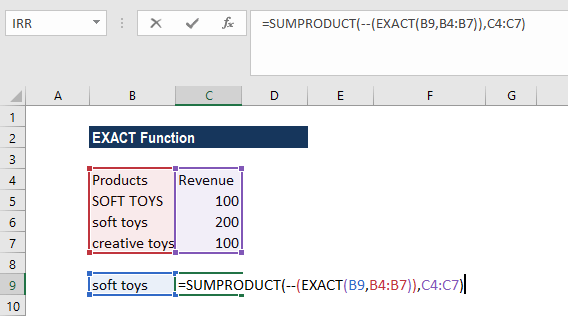The above formula will run a test on the values in column B, then convert the resulting TRUE/FALSE values to 1’s and 0’s. The reason we use a double negative is that it converts the resulting TRUE & FALSE values to 1’s and 0’s.

We will get the following result: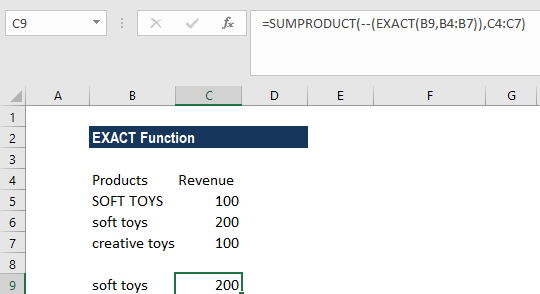Thanks for reading CFI’s guide to important Excel functions! By taking the time to learn and master these functions, you’ll significantly speed up your financial analysis. To learn more, check out these additional CFI resources:

• Excel Functions for Finance